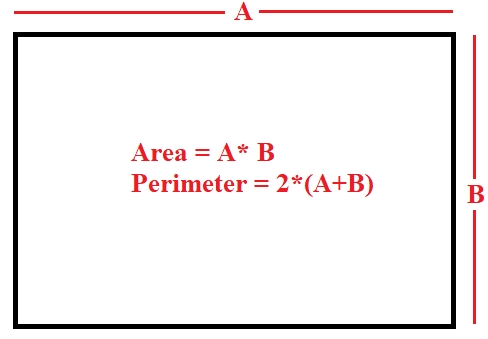# C Program for Area And Perimeter Of Rectangle

Given a length and breadth of a rectangle we have to find its area and Perimeter.

Rectangle is 2-D figure containing four sides and four angles of 90 degree each. All the sides of rectangle are not equal only the opposite sides of a rectangle are equal. The diagonals in a rectangle also are of the same length.

Below is a diagrammatic representation of rectangle.Here A represents the breadth and B represents length of the rectangle.

To find the Area of a rectangle the formula is: Length x Breadth

And Perimeter of a rectangle is − 2 x (Length+Breadth).

## Examples

Input: 20 30
Output: area of rectangle is : 600
perimeter of rectangle is : 100

## Algorithm

START
In Function int area(int a, int b)
Step 1 -> Declare an integer ‘area’ and store a * b in it
Step 2 -> Return area.
In Function int perimeter(int a, int b)
Step 1-> Declare an integer ‘perimeter’ and store 2*(a + b) in it
Step 2-> Return perimeter
In int main()
Step 1 -> Declare two integers ‘length’, ‘breadth’
Step 3 -> Print perimeter(length, breadth);
STOP

## Example

#include<stdio.h>
//function to calculate area
int area(int a, int b) {
int area = a * b;
return area;
}
//function to calculate perimeter
int perimeter(int a, int b){
int perimeter = 2*(a + b);
return perimeter;
}
int main(){
int length= 20;
}
area of rectangle is : 600
perimeter of rectangle is : 100